Chapter 11.7, Problem 58E

Chapter
Section
Textbook Problem

# MatchingIn Exercises 71–76, match the equation (written in terms of cylindrical or spherical coordinates) with its graph. [The graphs are labeled (a), (b), (c), (d), (e), and (f).](a)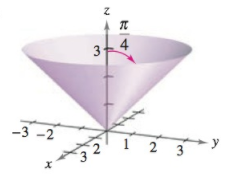(b)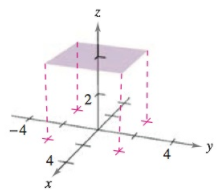(c)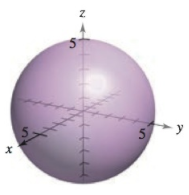(d)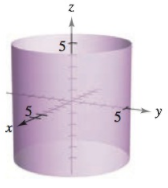(e)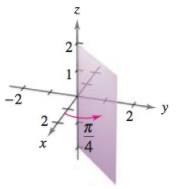(f)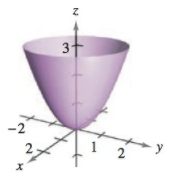θ = π 4

To determine
For the given graphs, find the appropriate graph for the equation θ=π4.

Explanation

Given:

The following provided graphs are:

Explanation:

Convert cylindrical coordinates to rectangular coordinates.

Given is a cylindrical equation,

As the equation is

θ=π4

We know,

tanθ=yx

Put values,

tan(π4

### Still sussing out bartleby?

Check out a sample textbook solution.

See a sample solution

#### The Solution to Your Study Problems

Bartleby provides explanations to thousands of textbook problems written by our experts, many with advanced degrees!

Get Started